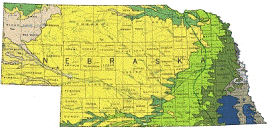## US Geological Survey## USGS Staff -- Published Research

#### Date of this Version

1992

Published in Hydraulic Engineering: Saving a Threatened Resource—In Search of Solutions: Proceedings of the Hydraulic Engineering sessions at Water Forum ’92. Baltimore, Maryland, August 2–6, 1992. Published by American Society of Civil Engineers.

#### Abstract

In 1946, Vedernikov developed a stability criterion for uniform flow in open channels. A global parameter proposed by Vedernikov has been widely accepted as a measure of flow stability. Recognizing the significance of the role of the parameter playing in the stability of open-channel flows, hydraulicians have thenceforth called it the Vedernikov number. Alternatively, the Vedernikov number may be considered as an extended form of the Froude number because it equals the Froude number mUltiplied by an exponent of the hydraulic radius in the uniform-flow formula and a shape factor of channel section. A stability criterion can thus be formulated using the Vedernikov number as follows: When the Vedernikov number exceeds unity, disturbances created in the flow will amplify, but when it is less than unity, some (not all) disturbances will attenuate. The specific state of flow with Vedernikov's number equal to unity thus represents a limiting case of neutral stability. The Froude number corresponds to this neutral stability may be referred to as the stability limit for the Froude number (or often called the critical Froude number). Therefore, if the Froude number instead of the Vedernikov number is used in the stability criterion, the stability limit for the Froude number that has yet to be determined from the expression of the Vedernikov number replaces Vedernikov's unity. There are numerous ways to derive the stability criterion using either the Vedernikov number or the Froude number. In either case, the stability criterion can vary with the absolute velocity of disturbance waves, which in turn depends on the velocity distribution of flow in channels. This paper is aimed to compare the existing methods in the formulation of the stability criterion, thereby assessing the importance of the role that the velocity distribution plays in the stability of open-channel flows.

COinS